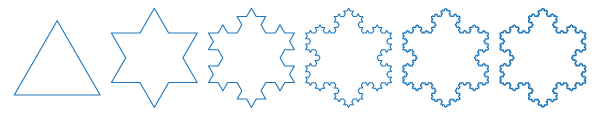# Year 7

## Number and Algebra

### Multiplication and division of fractions

The word 'fraction' comes from the Latin word fractus, meaning 'broken into pieces'. Fractions have been the standard method for representing parts of a whole for thousands of years. The ancient Egyptians and Greeks used unit fractions, and were concerned with ratio. There is evidence that the Chinese also made use of fractions at a very early date. It is believed that the version of fractions we use today is derived from the Hindu representation, though the Arabs introduced the 'bar'. This representation emerged in Western Europe in the late Middle Ages.

Competence and confidence with multiplying and dividing fractions is essential for everyday use. It is also very important in the manipulations of algebraic expressions.The famous Koch snowflake (pictured) is formed by repeated multiplication. One starts with an equilateral triangle. The side length of the triangles in the second stage is $$\dfrac{1}{3}$$ that of the first stage; the side length of the triangles in the third stage is $$\dfrac{1}{3}$$ that of the second stage, and so on.

So if the original triangle has a length of 1 unit, the triangles in the sixth version have side length $$1 × \dfrac{1}{3} × \dfrac{1}{3} × \dfrac{1}{3} × \dfrac{1}{3} × \dfrac{1}{3} = (\dfrac{1}{3})^5$$.